## Simple harmonic motion

11-17-99

Sections 10.1 - 10.4

### The connection between uniform circular motion and SHM

It might seem like we've started a topic that is completely unrelated to what we've done previously; however, there is a close connection between circular motion and simple harmonic motion. Consider an object experiencing uniform circular motion, such as a mass sitting on the edge of a rotating turntable. This is two-dimensional motion, and the x and y position of the object at any time can be found by applying the equations:The motion is uniform circular motion, meaning that the angular velocity is constant, and the angular displacement is related to the angular velocity by the equation: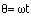Plugging this in to the x and y positions makes it clear that these are the equations giving the coordinates of the object at any point in time, assuming the object was at the position x = r on the x-axis at time = 0: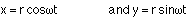How does this relate to simple harmonic motion? An object experiencing simple harmonic motion is traveling in one dimension, and its one-dimensional motion is given by an equation of the form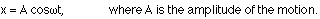The amplitude is simply the maximum displacement of the object from the equilibrium position.

So, in other words, the same equation applies to the position of an object experiencing simple harmonic motion and one dimension of the position of an object experiencing uniform circular motion. Note that thein the SHM displacement equation is known as the angular frequency. It is related to the frequency (f) of the motion, and inversely related to the period (T):The frequency is how many oscillations there are per second, having units of hertz (Hz); the period is how long it takes to make one oscillation.

### Velocity in SHM

In simple harmonic motion, the velocity constantly changes, oscillating just as the displacement does. When the displacement is maximum, however, the velocity is zero; when the displacement is zero, the velocity is maximum. It turns out that the velocity is given by: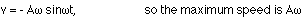### Acceleration in SHM

The acceleration also oscillates in simple harmonic motion. If you consider a mass on a spring, when the displacement is zero the acceleration is also zero, because the spring applies no force. When the displacement is maximum, the acceleration is maximum, because the spring applies maximum force; the force applied by the spring is in the opposite direction as the displacement. The acceleration is given by: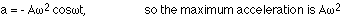Note that the equation for acceleration is similar to the equation for displacement. The acceleration can in fact be written as: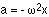All of the equations above, for displacement, velocity, and acceleration as a function of time, apply to any system undergoing simple harmonic motion. What distinguishes one system from another is what determines the frequency of the motion. We'll look at that for two systems, a mass on a spring, and a pendulum.

### The frequency of the motion for a mass on a spring

For SHM, the oscillation frequency depends on the restoring force. For a mass on a spring, where the restoring force is F = -kx, this gives: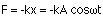This is the net force acting, so it equals ma: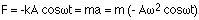This gives a relationship between the angular velocity, the spring constant, and the mass: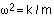### The simple pendulum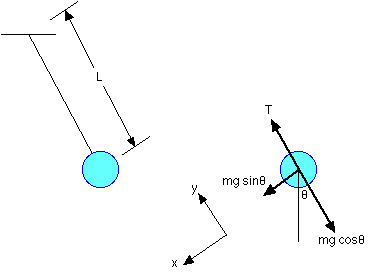A simple pendulum is a pendulum with all the mass the same distance from the support point, like a ball on the end of a string. Gravity provides the restoring force (a component of the weight of the pendulum).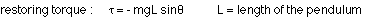Summing torques, the restoring torque being the only one, gives:For small angular displacements :So, the torque equation becomes: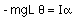Whenever the acceleration is proportional to, and in the opposite direction as, the displacement, the motion is simple harmonic.

For a simple pendulum, with all the mass the same distance from the suspension point, the moment of inertia is: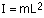The equation relating the angular acceleration to the angular displacement for a simple pendulum thus becomes: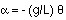This gives the angular frequency of the simple harmonic motion of the simple pendulum, because: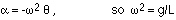Note that the frequency is independent of the mass of the pendulum.